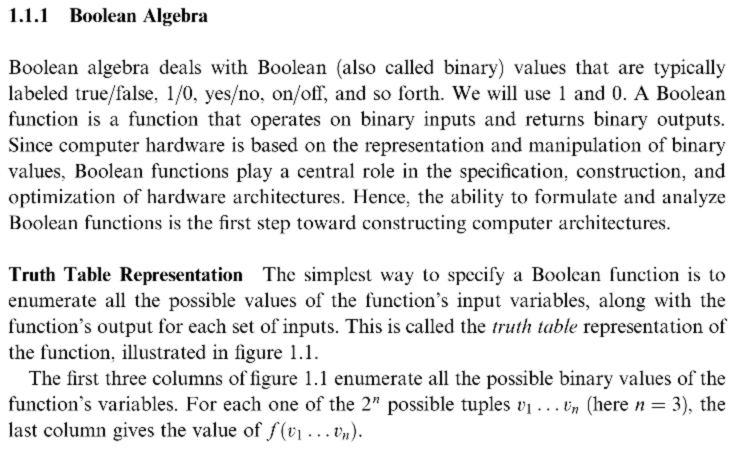# Boolean Logic Truth TableClassicListThreaded5 messagesOpen this post in threaded view
|

## Boolean Logic Truth Table

 In chapter 1, Truth Table Representation, there is a sentence: "For each one of the 2n possible tuples v1 ... vn (here n ¼ 3)" What exactly does this mean? I see there is x, y, z and then the output on the right. 2^n , there are 3 values , what is being raised to the nth power. What is 1/4 3. Help please what is this saying?
Open this post in threaded view
|

## Re: Boolean Logic Truth Table

 Administrator Looks like character set translation problems. Which text is this in? (Give the URL if you can.) Read it as "For each one of the 2 to the nth power possible tuples v1 ... vn (here n is equal to 3)" There are 3 inputs to the function, so x can be 0 or 1, y can be 0 or 1, and z can be 0 or 1. This gives 2^3 possible combinations of x, y, and z.
Open this post in threaded view
|

## Re: Boolean Logic Truth Table

 LINK as requestedOk, I get it there are 8 possible combinations. Looking at the chart. Thanks for helping me understand a bit more. However, when I use an online calculator to calculate the permutations of 2 numbers, 3 at a time. Tells me N has to be bigger than R. I guess the calculator was not set up to calculate permutations of boolean numbers? I know this is off topic, but I'm weird like that.
 Administrator pimaths66 wrote LINK as requested This PDF displays correctly for me.What are you using as a PDF viewer? Ok, I get it there are 8 possible combinations. Looking at the chart. Thanks for helping me understand a bit more. However, when I use an online calculator to calculate the permutations of 2 numbers, 3 at a time. Tells me N has to be bigger than R. I guess the calculator was not set up to calculate permutations of boolean numbers? I know this is off topic, but I'm weird like that. "Combinations" is being used in the text as the common language usage, not the mathematical probability sense. The online tool is correct. The definition of nPk is ``` n! -------- (n-k)! ```If n < k, then n−k factorial is undefined. Mathematically, the number of rows in a truth table is "counting". See http://mathworld.wolfram.com/CountingGeneralizedPrinciple.html.